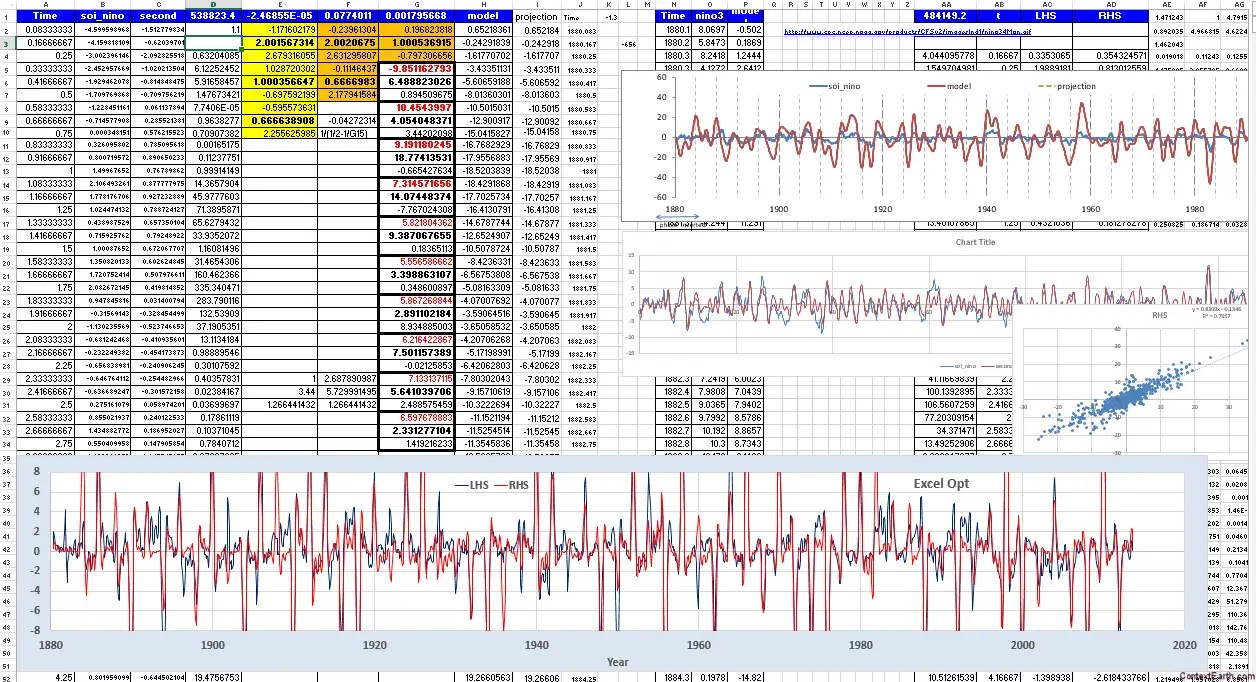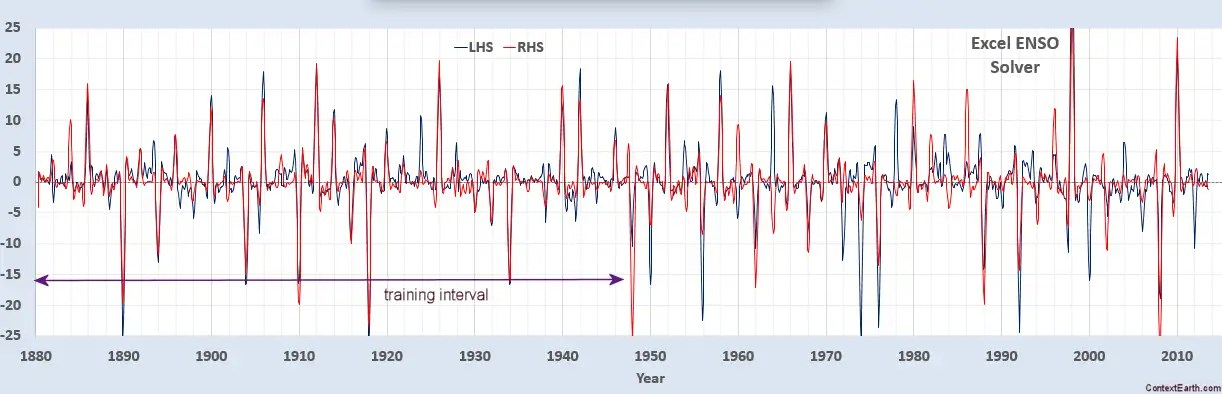# Solver vs Multiple Linear Regression for ENSO

In the previous ENSO post I referenced the Rajchenbach article on Faraday waves.

There is a telling assertion within that article:

“For instance, to the best of our knowledge, the dispersion relation (relating angular frequency ω and wavenumber k) of parametrically forced water waves has astonishingly not been explicitly established hitherto. Indeed, this relation is often improperly identified with that of free unforced surface waves, despite experimental evidence showing significant deviations”

What they are suggesting is that too much focus has been placed on natural resonances and the dispersion relationships within a free fluid volume. Whereas the forced response is clearly as important — if not more — and that the forcing will show through in the solution of the equations. I have been pursuing this strategy for a while, having started down the Mathieu equation right away and then eventually realizing the importance of the forced response, yet the Rajchenbach article is the first case that I have found made of what I always thought should be a rather obvious assumption. The fact that the peer-reviewers allowed the “astonishingly” adjective in the paper is what makes it telling. It’s astonishing in the equivalent sense that Rajchenbach & Clamond are pointing out that a pendulum’s motion will be impacted by a periodic push. In other words, astonishing in the sense that this premise should be obvious!

So, what I take as reinforcement from the article is that there has been a misguided focus on unconstrained or free fluid flow, as opposed to solving the dynamics of a Newtonian fluid within an enclosing container. Read through it and see if you come up with a similar understanding.

Further, what I am also finding is that in the cases of ENSO and QBO, the fundamental seasonality forcing does not jump out at you, but in fact is mixed into the stew and is not revealed by conventional methods. What you need is a good model of the physics and novel means of extracting the parameters from the dynamics, such as using the Mathieu equation for fluid dynamics — as Rajchenbach suggests and I have been advocating for ENSO. That’s why it hasn’t been obvious over the years, and the continuing claim being made that ENSO (by Tsonis) and QBO (by Lindzen) are driven by natural/chaotic resonances lives on …

I have off-and-on used the Excel Solver to optimize model fits and decided to try it again to supplement the multiple linear regression approach that I have been using.  The regression is very fast for specific parameters but a Solver can explore more possibilities over a longer training time. The Mathieu equation that I have been using reflects that of Rajchenbach’s formulation. Rajchenbach considers the Mathieu modulation itself a forcing, which is entirely valid, but I add a RHS term forcing g(t) which is supported by references on sloshing behavior by Frandsen and by Faltinsen.$f''(t) + omega_0^2 (1 + F cos(pi t))f(t) = g(t)$

The Mathieu modulation term shown in the above equation is a biennial period, but I added Hill terms for annual, semiannual, and 2/3 of a year in addition to the 2-year period. The last factor of 2/3 is a result of beating a 2-year period against the annual period as shown in the previous post. Not a problem adding these terms as the Solver should be able to sort them out and place a small magnitude on the coefficient if it’s not a strong factor. For the g(t) terms I applied the strong 6.5, 14, 18.6 year angular momentum (Chandler wobble) terms and the seasonally aliased tropical, anomalous, etc tidal terms as seeded input for the Solver.

So that the Excel input looked approximately like the following inset (similar to that described here) , where the A column is time, the B column contains the ENSO values, the C column is the ENSO 2nd-derivative, and the rest E,F,G terms are parameters:

LHS
\$C4+(\$G\$1+ \$E\$2*COS(2*PI()/\$E\$3*\$A4-\$E\$4)+ \$E\$5*COS(2*PI()/\$E\$6*\$A4-\$E\$7)
+\$E\$8*COS(2*PI()/\$E\$9*\$A4-\$E\$10))*(\$B4)

RHS
(\$F\$1+\$G\$2*COS(2*PI()/\$G\$3*\$A4-\$G\$4)+\$F\$2*COS(2*PI()/\$F\$3*\$A4-\$F\$4)+\$F\$5*COS(2*PI()/\$F\$6*\$A4-\$F\$7))*
(\$G\$5*COS(2*PI()/\$G\$6*\$A4-\$G\$7)+\$G\$8*COS(2*PI()/\$G\$9*\$A4-\$G\$10)
+\$G\$11*COS(2*PI()/\$G\$12*\$A4-\$G\$13)+\$G\$14*COS(2*PI()/\$G\$15*\$A4-\$G\$16)
+\$G\$17*COS(2*PI()/\$G\$18*\$A4-\$G\$19)+\$G\$20*COS(2*PI()/\$G\$21*\$A4-\$G\$22)
+\$G\$23*COS(2*PI()/\$G\$24*\$A4-\$G\$25)+\$G\$26*COS(2*PI()/\$G\$27*\$A4-\$G\$28)
+\$G\$29*COS(2*PI()/\$G\$30*\$A4-\$G\$31)+\$G\$32*COS(2*PI()/\$G\$33*\$A4-\$G\$34))

This is actually not as complicated as it looks, and after entering the set and initiating a run, the Solver locks in strongly to the Mathieu terms and barely budges from the periods of 1/2, 2/3, 1, and 2 years. The RHS terms adjust a bit from the seed but not much more than a few percent. The optimizing target is a hybrid metric of correlation coefficient and scaled error.Fig. 1: Spreadsheet view. Note that the cells highlighted in yellow (bright=LHS, darker=RHS) barely move from the nominal annual values after the fit completes. The red values are near the non-annually-aligned forced terms found via a multiple linear regression approach.Fig. 2: Full fit, not including 1/2 year term. The yellow regions are discrepancy regions. The peaks are lopped off to allow one to focus on the subtle feature matching in the weaker amplitudes. The horizontal line between 1980 and 1996 is the phase inverted interval .Fig. 3: Absolute error between model and wave equation transformed ENSO data.Fig. 4: Training up to 1947, showing excellent validation up to current date.Fig. 5: Adding the 1/2 year term does not improve the correlation coefficient much but does add sharper fine structure matching.

As I have mentioned in a previous post (pending link), there is a degree of “artificial” correlation that occurs with the Mathieu wave equation fitting algorithm, since the ENSO signal is being modulated by a known waveform on the LHS and so that can be recovered to some extent on the RHS. However, the correlation could just as easily be anti if the modulation happened to not align with the +/- excursion of the data. And yet we see very little of anti-correlated regions in Figure 2 — and considering how noisy the ENSO signal is, the Solver fitting algorithm generates a strong match between data and model.

This is also a very simple model to set up and anybody with proficiency with Excel can duplicate these results. It definitely agrees with the previous regression approach I have been using, but this Solver was also able to identify the strong 2/3 year modulation that is predicted by non-linear period doubling from annual to biennial (note that a 1/3 year period caused by nonlinear interactions between 1 and 1/2 year periods might also be relevant, but I haven’t added that yet).

In review, the important observational premises to invoke in this model are:

1. A biennial mode is operational
2. A phase inversion occurs between 1980 and 1996, justified by metastability of the biennial model with respect to even and odd starting years.
3. Mathieu equation formulation for sloshing behavior
4. A right-hand-side (RHS) forcing due to known angular momentum and gravity terms, with additional seasonal aliasing

Compare these four premises to the four observational premises made for QBO and you can see how easily one can get stymied in arriving at a complete physical model. None of these are plainly obvious from the research literature on ENSO and QBO. But when the right physics gets complemented with the right math, the results can be quite promising, if not “astonishingly not been explicitly established hitherto“.

## 9 thoughts on “Solver vs Multiple Linear Regression for ENSO”

1.Dennis Coyne

Very nice. Could we combine this with CSALT, a set of shock models for carbon emissions, a simple Bern Model for the carbon cycle, and possibly QBO as well could be added to CSALT along with your QBO model to get a simple climate model.

Very cool.

Like

•admin

Dennis,
Of course it could be done, but I am less enthusiastic about that aspect in the near term. This is more science than the dogtrack. I am seeing a few other people parading their ENSO model forecasts around without the slightest idea of what physics they are invoking. They are essentially heuristic models as I mentioned at the end of the post above.

I really don’t want to be associated with them. They will fall hard when their heuristics don’t work out as they expect. So right now I am looking more for substantiation of the model.

Like

•Dennis Coyne

One possibility would be to find what works over the interval where you have good data and then run it back to 1750 where we have fossil fuel and land use change estimates (though only land temperature data going back that far, though Marcott et al 2013 could be used for a rough estimate from 1750 to 1850 for Global land ocean temperatures).

That might give some indication for a CSALT type simple model.

I thought it would be interesting, oh well.

Like

•Dennis Coyne

Hi WHT,

As far as the dogtrack, I am less interested in predicting exact temperatures for any year. I am interested in the general trend for say 10 year average temperatures, so climate not weather. Weather cannot be predicted (yet) very far in advance, the fluid dynamics is too complex for that.

I am interested in applying your work (Shock model, QBO, ENSO) in combination with a simple carbon model (Bern Model from Joos et al 2013) to show what is likely to happen based on reasonable fossil fuel estimates (likely between 1000 and 1500 Pg of total carbon emissions).

Like

2.admin

Dennis,
Appreciate your enthusiasm, but for the next month I have to focus on the physics since I’ll be presenting this research at the AGU in December. It won’t do me any good forecasting projections for multiple years out as a means of defending the model. That will only provoke eye-rolling at a conference. Believe me that this physics is a lot more slippery than the oil depletion models where the projections are no sweat to discuss. We know oil projections won’t be spot on just because of human decision making. Whereas the climate models are pure physics and so have to work based on claims I have made of determinism. . So there is no benefit if you happen to get it correct, and only discreditation or dismissal if you get it wrong. Not a cut-and-dried decision to make.

I will probably look at it next year.

Like

•admin

Thanks, I think I have run across them.

That second paper is arguably wrong

“.. it is argued that the period of the QBO is mainly controlled by the magnitude of the gravity wave (GW) vertical fluxes of horizontal momentum (GWMF) forcing the QBO, while the QBO amplitude is mainly determined by the phase speeds of the GWs that make up this momentum flux. ”

I found that the period is controlled by the frequency of the nodal lunar tide.

Like

•admin

Also that paper doesn’t realize that ENSO does a phase reversal after 1980, so that is why the QBO correlation coefficient changes sign with respect to ENSO

They are also trying to compare ENSO correl coeff against QBO velocity, whereas much better to do it against QBO acceleration.

Like